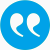#Fissler, Tobias, Holzmann, Hajo. 2022. Measurability of functionals and of ideal point forecasts.

BibTeX

## Abstract

The ideal probabilistic forecast for a random variable $Y$ based on an information set $\mathcal{F}$ is the conditional distribution of $Y$ given $\mathcal{F}$. In the context of point forecasts aiming to specify a functional $T$ such as the mean, a quantile or a risk measure, the ideal point forecast is the respective functional applied to the conditional distribution. This paper provides a theoretical justification why this ideal forecast is actually a forecast, that is, an $\mathcal{F}$-measurable random variable. To that end, the appropriate notion of measurability of $T$ is clarified and this measurability is established for a large class of practically relevant functionals, including elicitable ones. More generally, the measurability of $T$ implies the measurability of any point forecast which arises by applying $T$ to a probabilistic forecast. Similar measurability results are established for proper scoring rules, the main tool to evaluate the predictive accuracy of probabilistic forecasts.

## Tags

Press 'enter' for creating the tag

## Publication's profile

Status of publication Published WU Working/discussion paper, preprint English Measurability of functionals and of ideal point forecasts 2022 https://doi.org/10.48550/arXiv.2203.08635 AMS 2020 Classification: 62C99; 91B06

## Associations

People
Fissler, Tobias (Details)
External
Holzmann, Hajo (Philipps-Universität Marburg, Germany)
Organization
Institute for Statistics and Mathematics IN (Details)
Research areas (ÖSTAT Classification 'Statistik Austria')
1113 Mathematical statistics (Details)